28 March 2019

# Cobra parametric curve

### How to create parametric curves between two points

##### Paramteric curve: cobra_p2p()

Waveguides can be defined along a parametric curve where the position (x, y) of the spine of the curve is a function of parameter t: x(t), y(t).
The local width w(t) of the curve w is measured perpendicular to the spine, with the spine in the center.

The cobra in Nazca is a specific implementation of a smooth curve of constant width. See the following example for the point to point (p2p) cobra:

```# example created by Bright Photonics

import nazca as nd
import nazca.demofab as demo

p1 = (0, 10, 145)
p2 = (300, 300, -85)
demo.shallow.cobra_p2p(pin1=p1, pin2=p2).put()

nd.export_gds(filename='cobra1.gds')```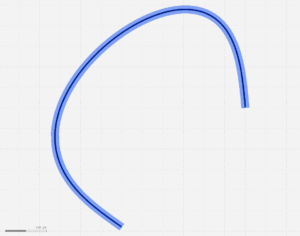Note that the cobra smoothly changes the radius, however, this by itself is not a condition for adiabaticity. The layout designer has to make sure that the change is gentle enough for the waveguide under consideration.

The cobra checks for a minimum radius, which is set in the xsection attribute ‘minimum_radius’. Nazca issues a warning if the radius drops under the minimum as demonstrated in the next example:

```# example created by Bright Photonics

import nazca as nd
import nazca.demofab as demo

for i in range (5):
s1 = demo.deep.strt(length=10).put(0, i*60)
s2 = demo.deep.strt(length=10).put(200, i*100)
demo.deep.cobra_p2p(pin1=s1.pin['b0'], pin2=s2.pin['a0']).put()
demo.shallow.cobra_p2p(pin1=s2.pin['a0'], pin2=s1.pin['b0']).put()

nd.export_gds(filename='cobra2.gds')```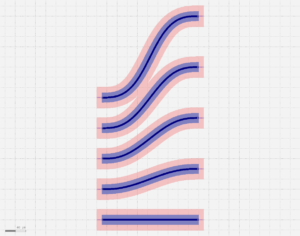The above example prints “minimum radius” warnings. The minimum radius is a property of the xsection used. It can be changed as indicated in the following example. When running the example the warnings adapt to the new setting(s).

```# example created by Bright Photonics

import nazca as nd
import nazca.demofab as demo

xs_shallow = nd.get_xsection('Shallow')

for i in range (5):
s1 = demo.deep.strt(length=10).put(0, i*60)
s2 = demo.deep.strt(length=10).put(200, i*100)
demo.deep.cobra_p2p(pin1=s1.pin['b0'], pin2=s2.pin['a0']).put()
demo.shallow.cobra_p2p(pin1=s2.pin['a0'], pin2=s1.pin['b0']).put()

nd.export_gds(filename='cobra2b.gds')```The cobra takes into account the curvature at the start and end of the curve via parameters radius1 and radius2. By default the cobra assumes no curvature, i.e. infinite radius (a straight guide), which is expressed in cobra by radius = 0.

The next example shows how to connect cobra with a continuous curvature to other guides.

```# example created by Bright Photonics

import nazca as nd
import nazca.demofab as demo

s1 = demo.deep.strt(length=100).put(0)
s2 = demo.deep.strt(length=100).put(200, 200)
demo.deep.cobra_p2p(pin1=s1.pin['a0'], pin2=s2.pin['a0']).put()

R2 = 100
b1 = demo.shallow.bend(angle=-45).put(0)
demo.shallow.cobra_p2p(pin1=b1.pin['a0'], pin2=b2.pin['a0'],

nd.export_gds(filename='cobra3a.gds')```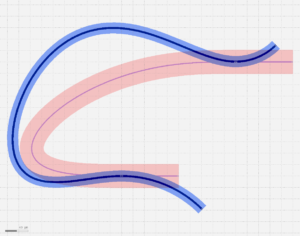The straight-to-bend offset is automatically applied to the cobra ends.

This is more explicitly visible in connections between the cobra and waveguides of different curvature at the connections:

```# example created by Bright Photonics

import nazca as nd
import nazca.demofab as demo

b1 = demo.shallow.strt(length=100).put(0, 0)
demo.shallow.cobra_p2p(pin1=b1.pin['a0'], pin2=b2.pin['a0'],

nd.export_gds(filename='cobra3b.gds')```

It is possible to overrule the offset values by the offset1 and offset2 parameters for the start and end offset, respectively.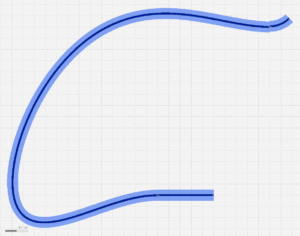In order to use cobra’s in your custom interconnects see the next example.
For completeness the example also includes the creation of a new xsection from scratch.

```# example created by Bright Photonics

import nazca as nd
from nazca.interconnects import Interconnect

# create layers:

# create a xsection and set some of its properties:
return 0

XS.width = 4.0
XS.os = os # straight-to-bend offset

# add layers to the xsection:

# create an interconnect object. It will get its properties from 'newXS':

# create a cobra with the interconnect:
b1 = ic.bend(angle=90).put(0)
ic.cobra_p2p(pin1=b1.pin['b0'], pin2=(400, 300, -85)).put()

# create a cobra with the interconnect, adapt the curvature:
b1 = ic.bend(angle=90).put(100, -50)

nd.export_gds(filename='cobra.gds')```

Use demofab_klayout_colors.lyp when viewing the gds in KLayout.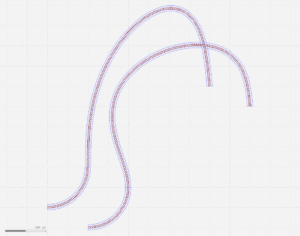## Related Tutorials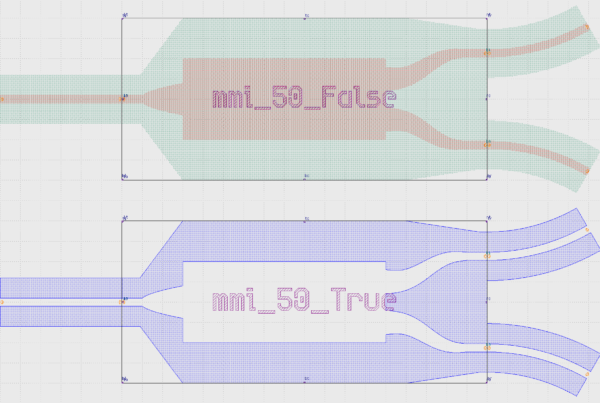In this example we show how to create an inverted MMI with custom interconnects.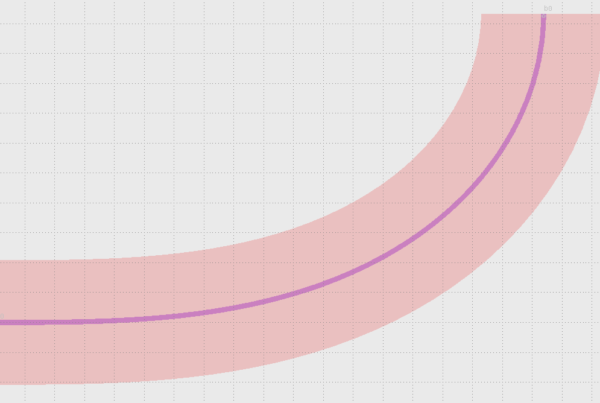In this example we show how to use Euler bends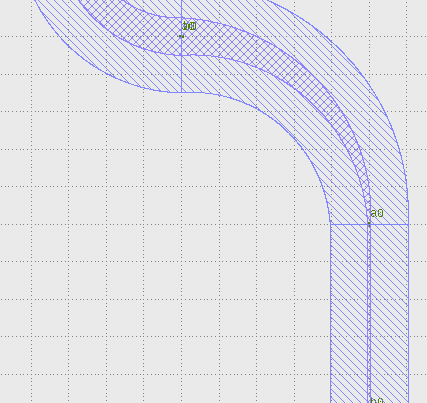In this example we show how to create a free form parametric curve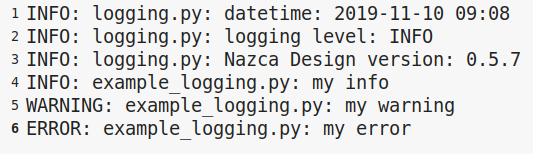In this example we show how to log your layout.